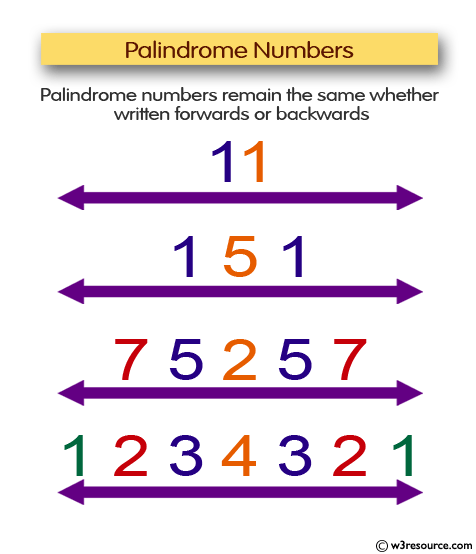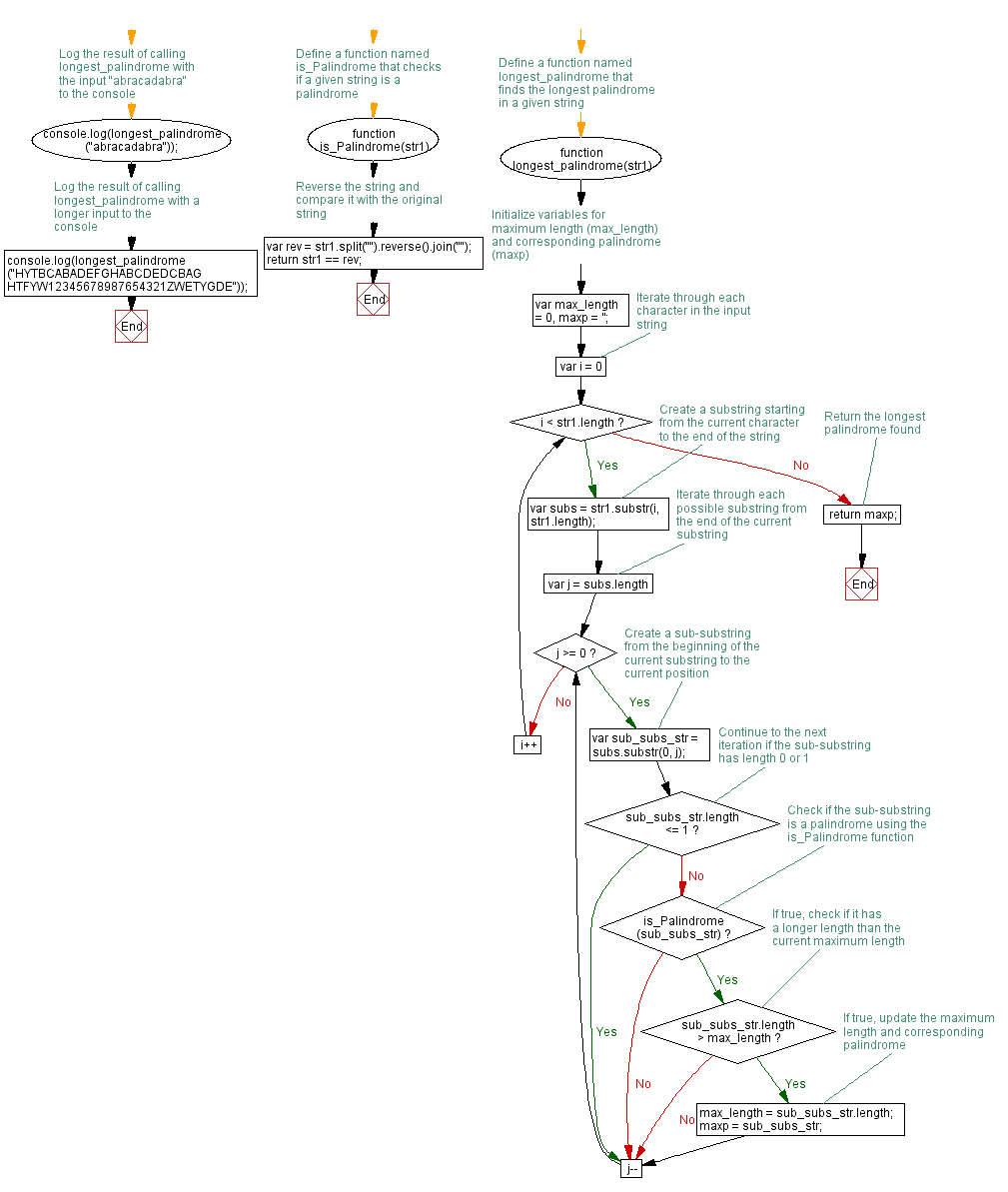# JavaScript: The longest palindrome in a specified string

## JavaScript Function: Exercise-27 with Solution

Write a JavaScript function that returns the longest palindrome in a given string.

Note: According to Wikipedia "In computer science, the longest palindromic substring or longest symmetric factor problem is the problem of finding a maximum-length contiguous substring of a given string that is also a palindrome. For example, the longest palindromic substring of "bananas" is "anana". The longest palindromic substring is not guaranteed to be unique; for example, in the string "abracadabra", there is no palindromic substring with length greater than three, but there are two palindromic substrings with length three, namely, "aca" and "ada".
In some applications it may be necessary to return all maximal palindromic substrings (that is, all substrings that are themselves palindromes and cannot be extended to larger palindromic substrings) rather than returning only one substring or returning the maximum length of a palindromic substring.

Pictorial Presentation:Sample Solution: -

HTML Code:

``````<!DOCTYPE html>
<html>
<meta charset="utf-8">
<title>Write a JavaScript function that returns the longest palindrome in a given string</title>
<body>

</body>
</html>
```
```

JavaScript Code:

``````function is_Palindrome(str1) {
var rev = str1.split("").reverse().join("");
return str1 == rev;
}

function longest_palindrome(str1){

var max_length = 0,
maxp = '';

for(var i=0; i < str1.length; i++)
{
var subs = str1.substr(i, str1.length);

for(var j=subs.length; j>=0; j--)
{
var sub_subs_str = subs.substr(0, j);
if (sub_subs_str.length <= 1)
continue;

if (is_Palindrome(sub_subs_str))
{
if (sub_subs_str.length > max_length)
{
max_length = sub_subs_str.length;
maxp = sub_subs_str;
}
}
}
}

return maxp;
}

```
```

Output:

```aca
12345678987654321
```

Flowchart:Live Demo:

See the Pen JavaScript - The longest palindrome in a specified string-function-ex- 27 by w3resource (@w3resource) on CodePen.

Improve this sample solution and post your code through Disqus

What is the difficulty level of this exercise?

﻿

## JavaScript: Tips of the Day

JavaScript: Implicit type coercion

```function sum(a, b) {
return a + b;
}

sum(1, '2');
```

JavaScript is a dynamically typed language: we don't specify what types certain variables are. Values can automatically be converted into another type without you knowing, which is called implicit type coercion. Coercion is converting from one type into another.

In this example, JavaScript converts the number 1 into a string, in order for the function to make sense and return a value. During the addition of a numeric type (1) and a string type ('2'), the number is treated as a string. We can concatenate strings like "Hello" + "World", so what's happening here is "1" + "2" which returns "12".

Ref: https://bit.ly/323Y0P6